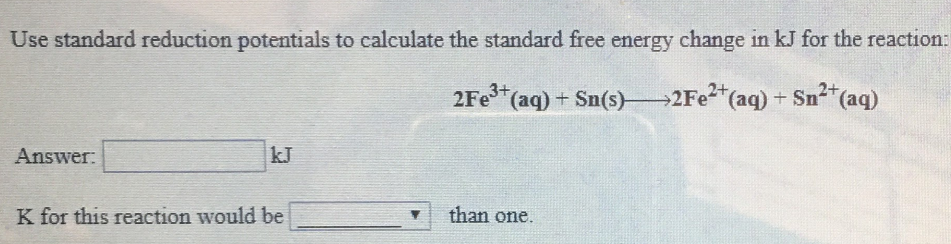# Use the standard reduction potentials to calculate the standard free energy change in kJ for the reaction: 2Fe^3+(aq) + Sn(s) → 2Fe^2+(aq) + Sn^2+(aq) answer: ____ kJ K for this reaction would be ____ than one.